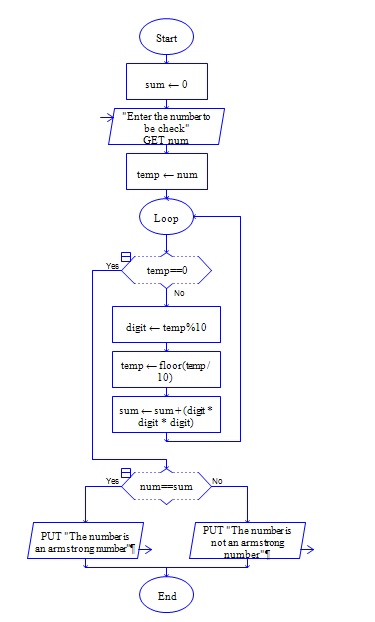# Find a number is Armstrong number or not using Python

Get a number and sum the digits of the number.

Sample Input 1:

Enter the number: 153

Sample Output 1:

Armstrong Number

Sample Input 2:

Enter the number: 213

Sample Output 2:

Not an Armstrong Number

#### Flow Chart Design#### Program or Solution

``` num = int(input("enter a number"))n = numcount = 0while n != 0:    count = count + 1    n = n // 10    n = numtotal = 0while n!= 0:    rem = n % 10    total = total + (rem**count)    n = n // 10if num == total:    print("Armstrong number")else:    print("Not an Armstrong number")     ```

#### Output#### Program Explanation

using a while loop, count the number of digits in a number

initialize total is equal to 0

calculate the remainder of a number by doing number % 10,  add the remainder count  to the total and divide the number by the 10 and repeat the above steps till number becomes zero.

Check total is equal to original number entered by user, if it is equal print Armstrong Number else Not an Armstrong Number.